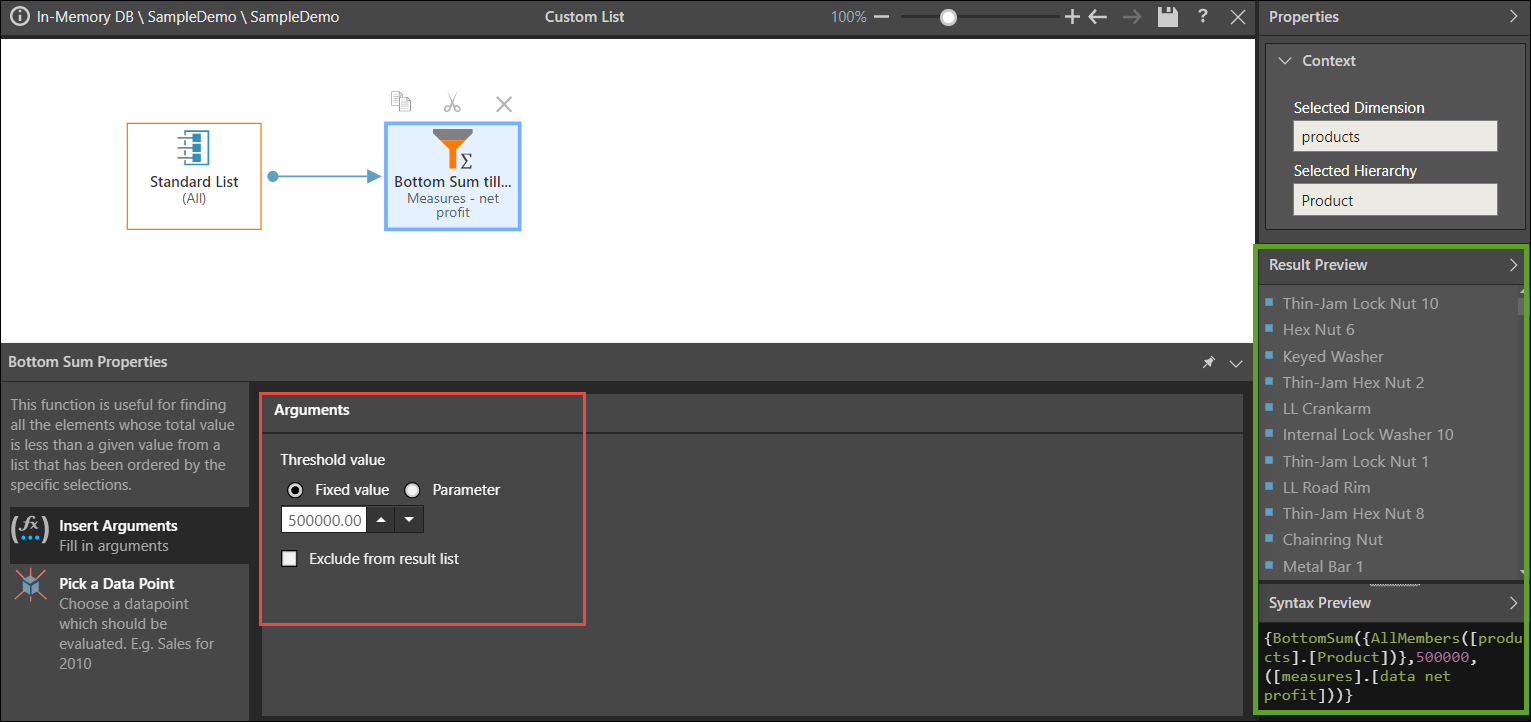# Bottom Sum

Returns a list of bottom member elements from derived from the given list, that sum to a specified value. The logic for deriving the list will use the optional numeric data point (which can be a fixed value or a parameter) if supplied. Otherwise, it uses the default selections for the model or the context in which the function was used.

#### Syntax & Usage

BottomSum([LIST], counter, optional numeric data-point)
• Create a list from which you want to derive the bottom sum list.
• Add the Bottom Sum block to the list, and set the counter from the Insert Arguments window under "Number of Items". This can be a static number or a numeric parameter.
• Select "Exclude from Result Set" if you want to remove the bottom sum elements from the list (as opposed to creating a list of bottom sum elements).

#### Example

This expression returns a list of the bottom Products by Net Profit that sum to 500,000; the given list is the Products hierarchy, and the Bottom Sum block is assigned a set value of 500,000 percent and Net Profit as the data point:

{BottomSum({AllMembers([products].[Product])},500000,([measures].[data net profit]))}